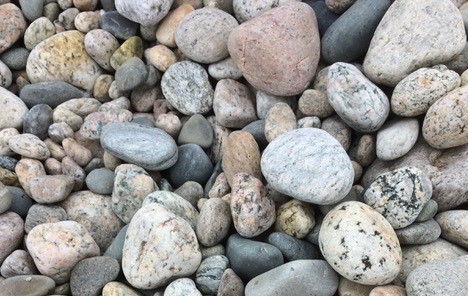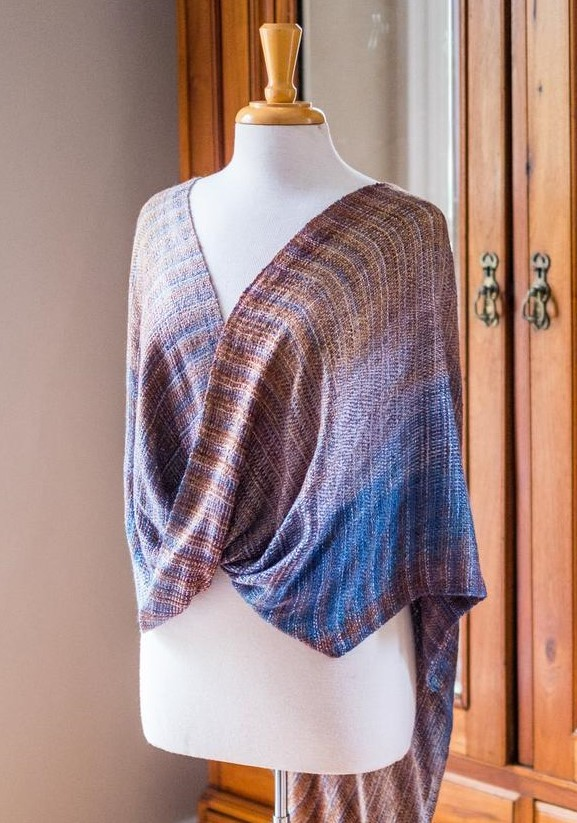Like   +1   in# Looking for some travel ideas this summer?

 table div table+table+table div table,table.module-2{width:53.77%;float:right;padding:0}table div table+table+table div table a{border:0 none;text-decoration:none}table div table+table+table div table img{width:100%!important;border:0 none;text-decoration:none}table div table+table+table div table td{width:100%;padding:0 0 20px 20px}/* styles */ For those of you in MA who are 62 and older, this senior parks pass is a great deal. A \$10 lifetime parking/entrance pass to the majority of the State Parks. You can buy it here. There are so many beautiful state parks in Massachusetts. Here's a list of some that you might want to visit this summer. Are any of your favorite places showing up? I love Halibut Point, Horseneck Beach and Nantasket Beach.
 table div table+table+table+table div table{width:100%;padding:0}table div table+table+table+table div table img{width:96.23%;padding:0;float:none}table div table+table+table+table div table td{width:100%;padding:0 1.88% 18px}/* styles */# Some places I hope to revisit this season...

 /* styles */ I'll be back at the Westport Winery this summer for sure—click here to learn more about it. Sit back, relax, and drink a glass while overlooking their beautiful vineyard. They have a great wine tasting tour, and if you're not feeling up to it, just buy some and take it home.
 table div table+table+table+table+table+table+table+table div table{width:100%;padding:0}table div table+table+table+table+table+table+table+table div table img{width:96.23%;padding:0;float:none}table div table+table+table+table+table+table+table+table div table td{width:100%;padding:0 1.88% 18px}/* styles */A diverse collection of rocks at Allens Pond's East Beach.Allens Pond sancturary wild flowers.

Allens Pond is another place on my list. There are acres of protected beach, fields, woodlands, ponds, and marsh that you can hike through.

Find out more about this beautiful Audubon reservation here. It's across the street from the Bayside Restaurant. Stop in when you're done with your hike for a lobsta' roll and cocktail!

## What are you doing this summer?

 table div table+table+table+table+table+table+table+table+table+table+table div table{width:100%;padding:0}table div table+table+table+table+table+table+table+table+table+table+table div table img{width:96.23%;padding:0;float:none}table div table+table+table+table+table+table+table+table+table+table+table div table td{width:100%;padding:0 1.88% 18px}/* styles */# I'm a-going fishin’!

Click to hear one of my all time favorite songs: Taj Mahal!
I'll be wearing my favorite shawls this summer—how will you accessorize? I've got just the piece for you at my Etsy stop. It will be open all summer with pieces you can throw-on for your next summer adventure.Blue, brown and cream rayon mobius shawl.
 table div table+table+table+table+table+table+table+table+table+table+table+table+table+table+table div table{width:100%;padding:0}table div table+table+table+table+table+table+table+table+table+table+table+table+table+table+table div table img{width:96.23%;padding:0;float:none}table div table+table+table+table+table+table+table+table+table+table+table+table+table+table+table div table td{width:100%;padding:0 1.88% 18px}/* styles */# Don't miss a thing.

Click the buttons below to get all the latest behind-the-scenes photos, thought provoking articles, and special offers. And thank you for sharing my newsletter.

 table div table+table+table+table+table+table+table+table+table+table+table+table+table+table+table+table+table div table{width:100%;padding:0}table div table+table+table+table+table+table+table+table+table+table+table+table+table+table+table+table+table div table table{padding:0;float:left!important;width:34.622%!important}table div table+table+table+table+table+table+table+table+table+table+table+table+table+table+table+table+table div table td{padding-left:6px;padding-right:6px}table div table+table+table+table+table+table+table+table+table+table+table+table+table+table+table+table+table div table table+table{float:left!important;width:34.622%!important}table div table+table+table+table+table+table+table+table+table+table+table+table+table+table+table+table+table div table table td,table div table+table+table+table+table+table+table+table+table+table+table+table+table+table+table+table+table div table table+table td{padding-left:0;padding-right:20px}table div table+table+table+table+table+table+table+table+table+table+table+table+table+table+table+table+table div table table+table+table{float:left!important;width:30.756%!important}table div table+table+table+table+table+table+table+table+table+table+table+table+table+table+table+table+table div table table+table+table td{padding-left:0;padding-right:0}/* styles */
 table div table+table+table+table+table+table+table+table+table+table+table+table+table+table+table+table+table+table div table{width:100%;padding:0}table div table+table+table+table+table+table+table+table+table+table+table+table+table+table+table+table+table+table div table img{width:96.23%;padding:0;float:none}table div table+table+table+table+table+table+table+table+table+table+table+table+table+table+table+table+table+table div table td{width:100%;padding:0 1.88% 18px}/* styles */# Have you forgotten all the ways to wear your mobius shawl?

## Check out this video and pass it along to your friends!

 table div table+table+table+table+table+table+table+table+table+table+table+table+table+table+table+table+table+table+table+table div table{width:100%;padding:0}table div table+table+table+table+table+table+table+table+table+table+table+table+table+table+table+table+table+table+table+table div table img{width:96.23%;padding:0;float:none}table div table+table+table+table+table+table+table+table+table+table+table+table+table+table+table+table+table+table+table+table div table td{width:100%;padding:0 1.88% 18px}/* styles */## Visit my website: hettyfriedmandesigns.com

Email: hetty.friedman@gmail.com Phone: 617.512.5344